# Stock market crash 2020 – the data says expect more drops

Updated: 06 April 2020

We have been in a high volatility market around the world due to the COVID-19, in the equity markets the volatility is higher when there is panic compared to when the euphoria is involved and this is known in the quantitative finance as the asymmetric in volatility (you can see an example in the implicit volatility smile from the option markets).

The index of volatility (using the SP500 index options) had its highest value since the financial crisis in 2008 and we have a down move of ~35% in SP500, ~40% in DAX and ~35% in Nikkei (among others) so, when are we going to see a change in the trend or at least a big bounce? that’s the purpose of this article and I assess that possibility using just linear regressions and moving averages, let’s begin: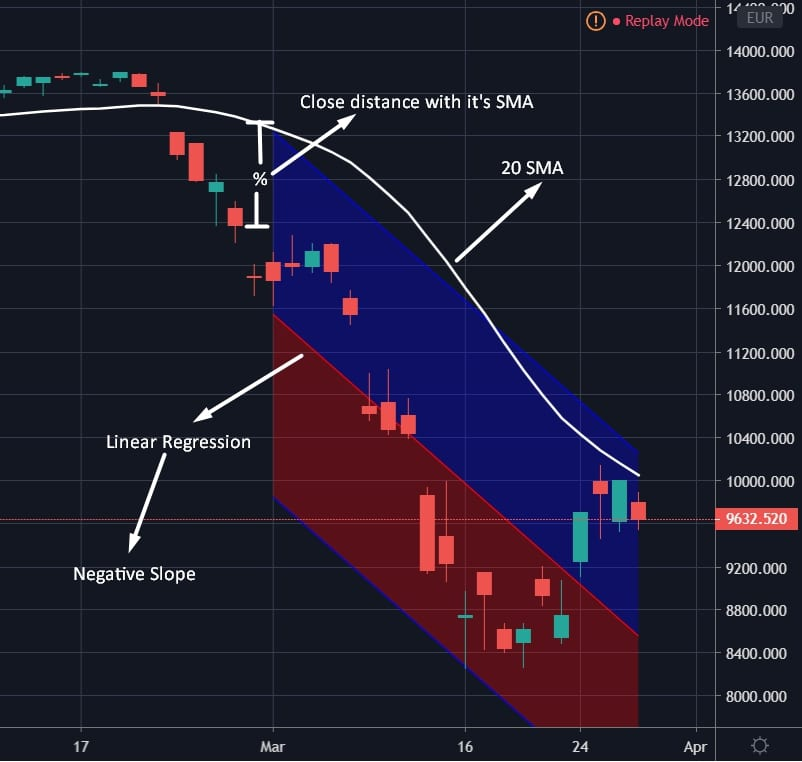We’re going to use the simple moving average as a measure of how long and how much the market has moved in one direction (down trend in this case), let’s assume that the down trend is present when the price is below its moving average (20 SMA in our first example) and the level of the down move as the maximum percentual change () between the price and its moving average, for example, the recent behavior in DAX show us that the highest percentual change between the price and the 20 period moving average is -25.82% (16-03-2020), after this day (till today) we’ve seen that this change has approached to zero (-1.59%, 27-03-2020). Another thing that we need to state before going deeper in the analysis is to define a bounce, for the purpose of this article let’s suppose that a bounce is when the price touches its moving average.

In this article, we’re going to use the slope of a linear regression to see how many bounces do we have before the price touches its moving average, sometimes the bounce is so fast and we not even had a chance to buy and sometimes we have several bounces because the market had more than one sell off. If we use the las 20 days to estimate a linear regression in the DAX Index, we may find that the price is too close to its 20 SMA but the regression’s slope is negative so, the bounce has been too fast.

Indeed, if we see how many days the price has been below the 20 SMA we find that the major indexes have more than 20 days (we’re going to use this threshold in the rest of the article) now the question is: How many times we see a positive slope after 20 days of sell off before the price touches its 20 SMA?

To answer this question, I used the historical data of the following indexes:

1. SP500
2. DAX
3. CAC40
4. FTSE
5. Nikkei
6. Hang Seng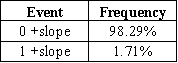98% of the time we do not even see a positive slope before the price touches its 20 SMA in other words, almost never. We can’t use this number without further analysis, this percentage covers those kind of healthy corrections without panic as the huge down movements too and we know that the emotions involved in both events are very different, at this point we should use the percentual distance that I mentioned above, it is not the same when you have a percentual distance of -20% compared to a -5% (see SPY on 06-06-2019), nowadays we have seen a huge percentual distance in all the indexes around the world, if we filter our table to those cases when the highest percentual distance is below -10% we get the following: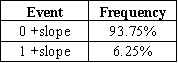Now the probability of getting a positive slope before the price touches the 20 SMA is a little bit higher but not too much.

### #1 Conclusion: There is a 93.75% probability that we see a fast bounce to the 20 SMA in the global indexes.

This information is not so useful right now because most of the indexes around the world have bounced too close to their 20 SMA but, what if we use a 200 SMA?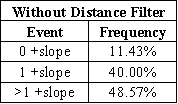We can see that there are other situations when the regression had two or more positive slopes (and there is a good chance that this event occurs), this means that the price consolidates after a down trend (all the time below the 200 SMA) and the slope turns positive, then we had another sell off and a new consolidation in order to see another positive slope, a good example is the following: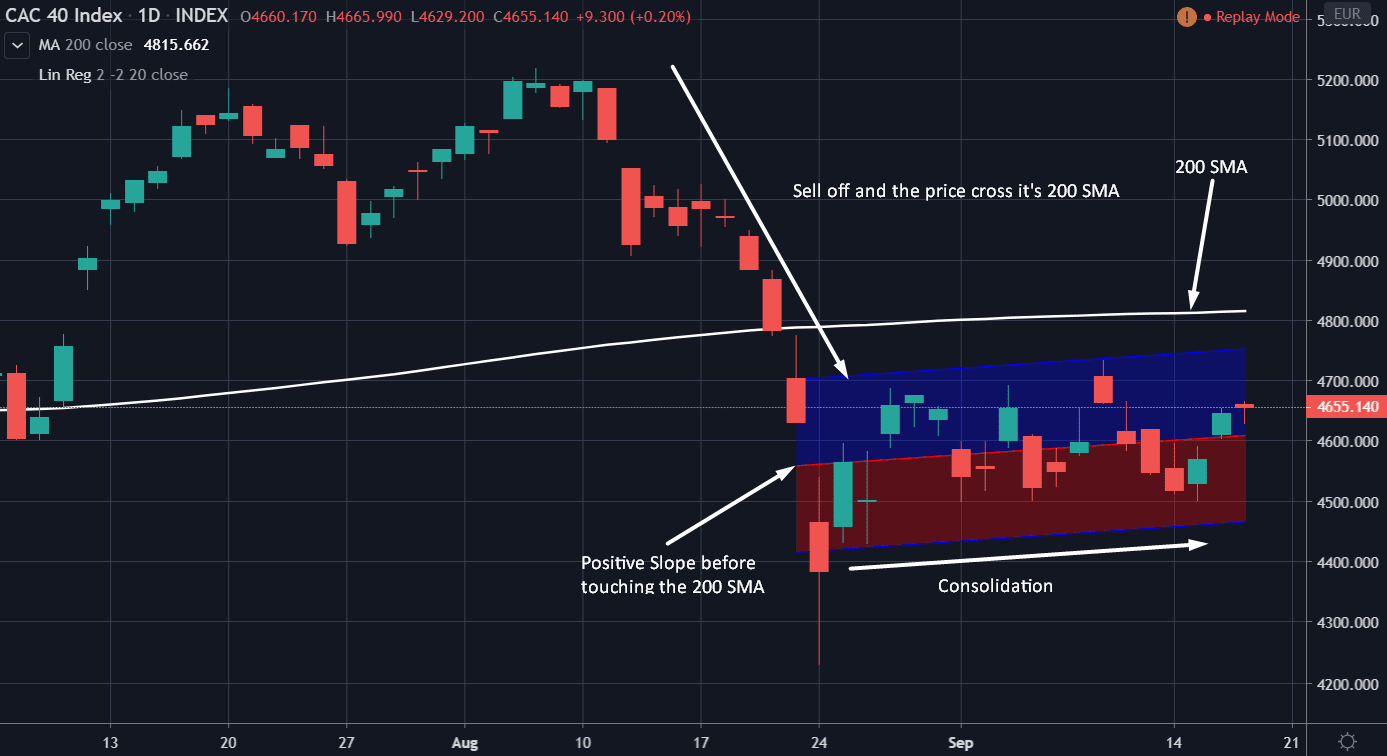This chart shows us the behavior of the CAC40 Index on September of 2015, we can see that after a sell off the price stays under its 200 SMA and consolidates, later we got a positive slope and we know that there is a 40% probability of getting just one positive slope before the price touches the 200 SMA, nevertheless this is not the case because the index had another sell off.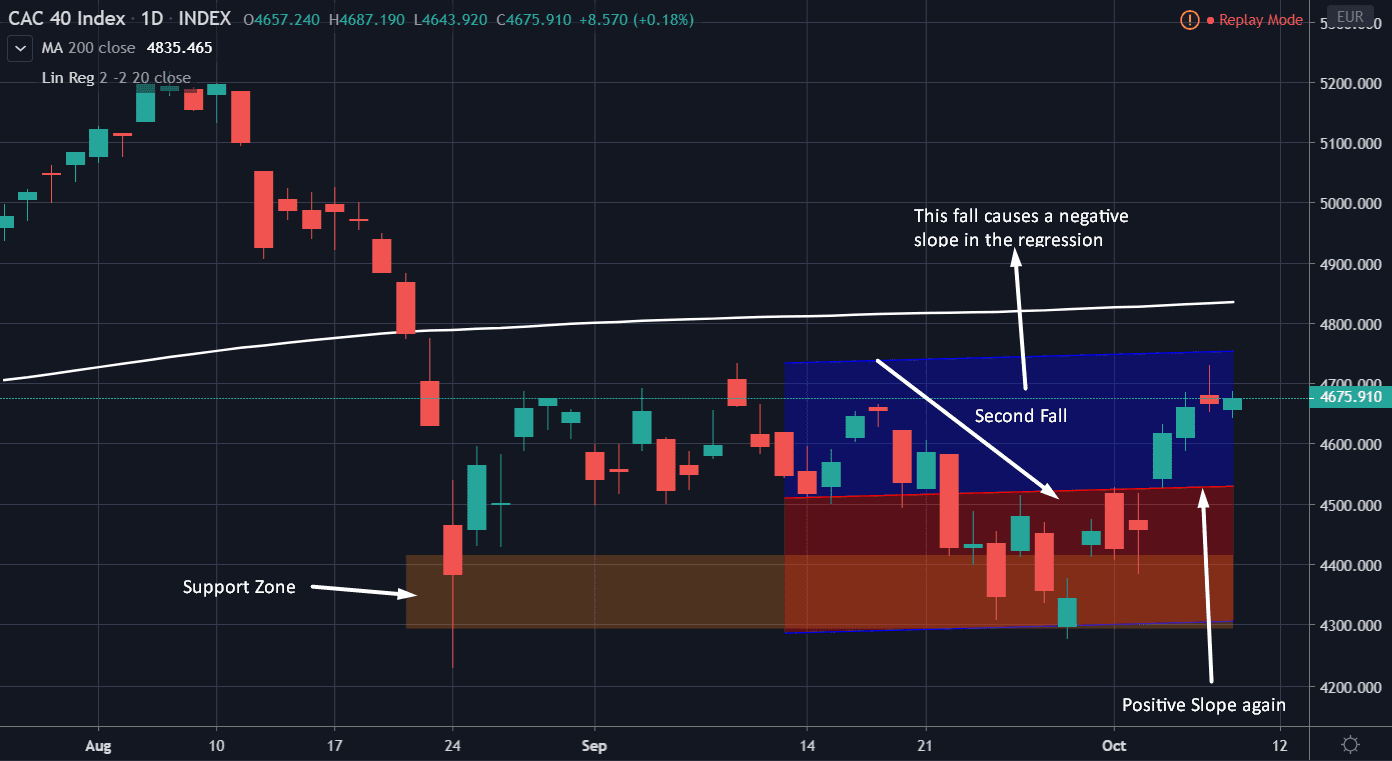So, we have two times when we see a positive slope before seeing the price crossing its 200 SMA. Would you implement this in your trading plan?

Let’s apply the distance filter again (percentual distance lower than -10% with respect to the 200 SMA), we could see that there is a higher probability of two or more positive slopes than less than two! Keep in mind that this table is more close to reality than the old one because again, the old table includes the normal corrections too.

### #2 Conclusion:  There is a good chance (67.44%) that the global indexes have another fall before touching their 200 SMA.

This information is useful because so many people are talking about when to buy stocks, trying to catch the recovery move if the COVID-19 would be under control, let’s see two examples: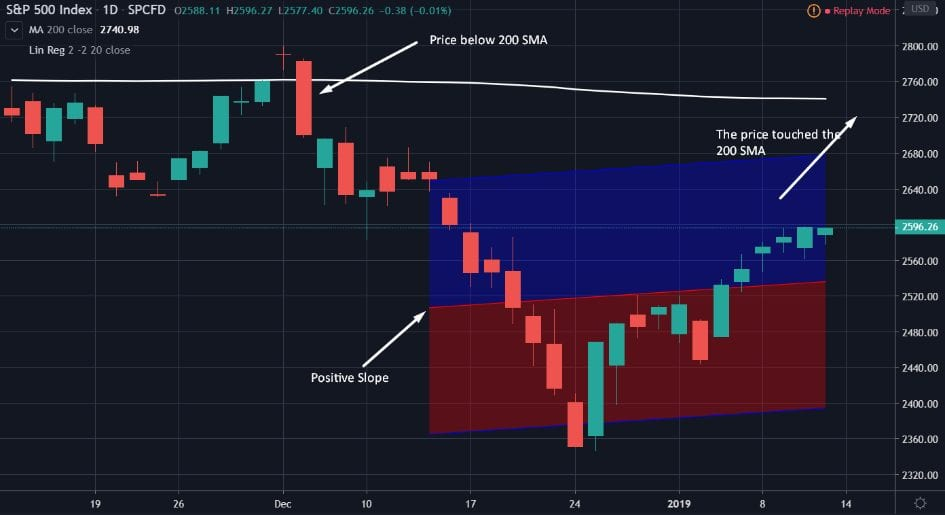The first example was in 11-01-2019, the SP500 index had a sell off due to the trade war between China and USA, but we saw a fast bounce to the 200 SMA without giving so many chances to catch that move.

This example corresponds to just one positive slope and now we know that this event has an occurrence probability of 20.93%

You can create a trading plan for this 20.93% of the cases but this plan should have a trade management plan in order to react to that 67.44% of the cases when we have two or more positive slopes (more falls).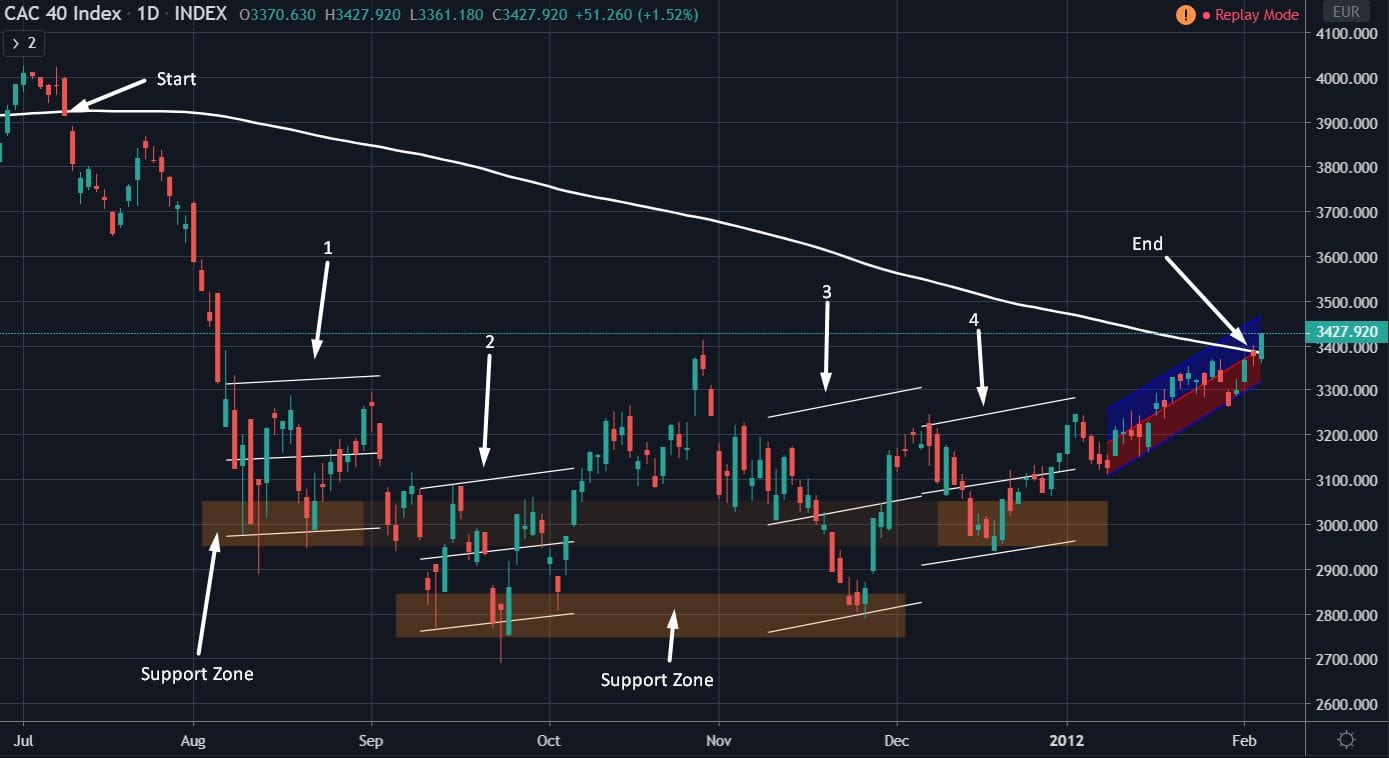The second example is interesting, because here we have several positive slopes (bounces), this behavior was over a long consolidation period after a huge fall. Psychologically is hard to accept that there is more chance of a second fall than a fast bounce and then some traders (and investors) tend to buy without a plan when the panic still there.

This example illustrates us how we should see a consolidation and we should expect more falls after a huge sell off in the market like we saw the past weeks, obviously these statistics were based on past data and this doesn’t mean that we’re going to see the exact same behavior but the pattern is there and the data is giving us this useful information, indeed, this is coherent with what so many experienced people are saying out there about waiting for another fall before the market find a bottom.